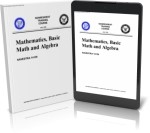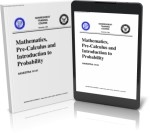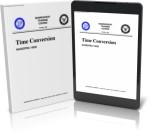## Navy Mathematics Manuals### 14139 Mathematics, Basic Math and Algebra

Provides a review of basic arithmetic and elementary algebra; includes fractions, decimals, percentages, exponents, radicals, logarithms; exercises in factoring polynomials, linear equations, ratio, proportion, variation, complex numbers and quadratic equations; presents brief introduction to plane figures, geometric construction, and trigonometry. - Pages: 304### 14141 Mathematics, Pre-Calculus and Introduction to Probability

Contains information on the following subjects: straight lines, conic sections, tangents, normals, slopes; introduction to differential and integral calculus; combinations and permutations; and introduction to probability. This course is general in nature and is not directed toward any specific specialty. - Pages: 384### 14252 Time Conversion

Assists enlisted and officer personnel of the United States Navy and Naval Reserve in acquiring the knowledge requisite to the computation of time. It uses two-dimensional charts and expanded narratives to explain both the global division and designation, and the processes and mathematical formulas used in the conversion of time. - Pages: 50